# Round function

Hello, I have a beast mode that calculates a % and I need it to round to 2 decimals.

I'm using the round function as described in the documentation:

CONCAT(ROUND(1-(COUNT(DISTINCT `nc_list`) / COUNT(DISTINCT `lot_number`)),2)*100, '%')

However, the result is being rounded to the whole number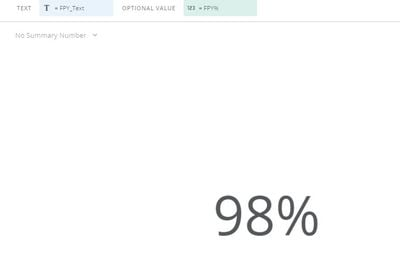I need it to, using the example above, display  97.91 instead of 98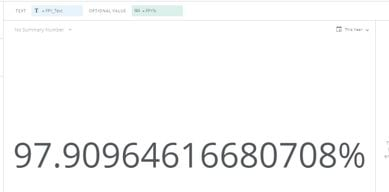What am I missing in my beast mode?

• You may not even need to round using a beast mode calculation. It seems like you are using a textbox chart, however for have you tried using the single value chart? It is a similar chart, and if you look in Chart Properties>General>Decimal Places, you will be able to select how many decimal places you would like to show.

•CoachHi @MartinB

The display options for the field can handle all of this for you.

You can change your beast mode to be:

`1-(COUNT(DISTINCT `nc_list`) / COUNT(DISTINCT `lot_number`))`

Then click on the Beast mode atop your chart to open the menu and select Data Format > Display as and select Percentage with 2 decimal places. You can then also tell it to multiply by 100.

**Did this solve your problem? Accept it as a solution!**
•CoachI know i'm late to the party, but @MartinB  it's just basic math.

CONCAT(ROUND(1-(COUNT(DISTINCT `nc_list`) / COUNT(DISTINCT `lot_number`)),2)*100, '%')

If you have 1/3 ( = .33333333) and you wrap that in round( 2) you'd get .33 so when you multiply by 100 you'd get 33.

if you wanted 33.33 then you do round(4).

Jae Wilson
Check out my 🎥 Domo Training YouTube Channel 👨‍💻

**Say "Thanks" by clicking the ❤️ in the post that helped you.
**Please mark the post that solves your problem by clicking on "Accept as Solution"

• You may not even need to round using a beast mode calculation. It seems like you are using a textbox chart, however for have you tried using the single value chart? It is a similar chart, and if you look in Chart Properties>General>Decimal Places, you will be able to select how many decimal places you would like to show.

•CoachHi @MartinB

The display options for the field can handle all of this for you.

You can change your beast mode to be:

`1-(COUNT(DISTINCT `nc_list`) / COUNT(DISTINCT `lot_number`))`

Then click on the Beast mode atop your chart to open the menu and select Data Format > Display as and select Percentage with 2 decimal places. You can then also tell it to multiply by 100.

**Did this solve your problem? Accept it as a solution!**
• Thanks @GrantSmith and @user096693 I'll try both approaches ?‍?

• @GrantSmith I tried your approach, however the details you explain apply only to the Optional Value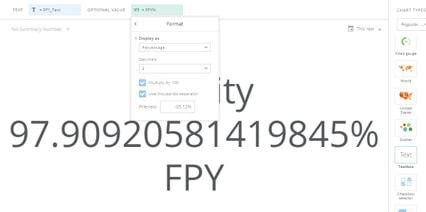• @user096693 thanks, your solution was exactly what I needed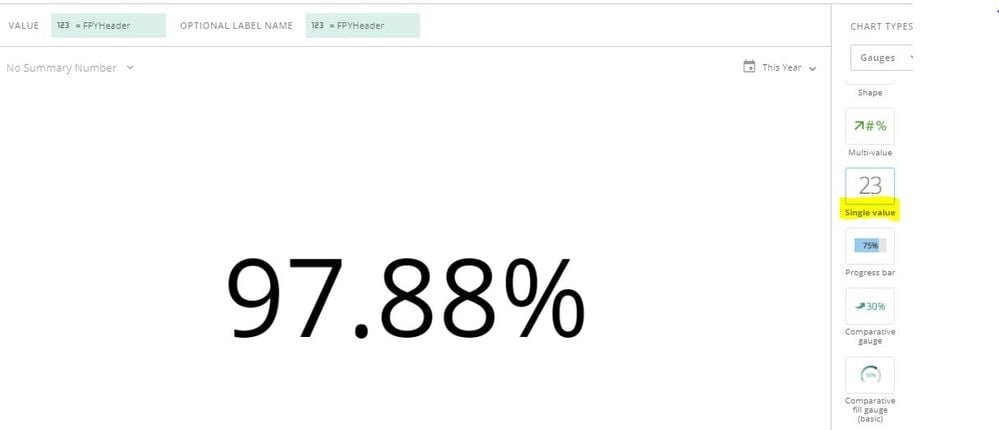•CoachI know i'm late to the party, but @MartinB  it's just basic math.

CONCAT(ROUND(1-(COUNT(DISTINCT `nc_list`) / COUNT(DISTINCT `lot_number`)),2)*100, '%')

If you have 1/3 ( = .33333333) and you wrap that in round( 2) you'd get .33 so when you multiply by 100 you'd get 33.

if you wanted 33.33 then you do round(4).

Jae Wilson
Check out my 🎥 Domo Training YouTube Channel 👨‍💻

**Say "Thanks" by clicking the ❤️ in the post that helped you.
**Please mark the post that solves your problem by clicking on "Accept as Solution"
• Silly me, this formula made the difference. Thanks @jaeW_at_Onyx

CONCAT(ROUND(1-(COUNT(DISTINCT `nc_list`) / COUNT(DISTINCT `lot_number`)),4), '%')

Still, had some trouble when adding strings either at the beginning or the end of the formula pasted above...until this I hit jackpot:

CONCAT('Quality ', CONCAT(ROUND(100*1-(COUNT(DISTINCT `nc_list`) / COUNT(DISTINCT `lot_number`)),2), '% FPY'))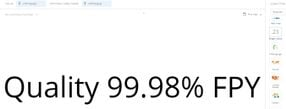Thanks to all, this is the solution I was looking for.

This discussion has been closed.